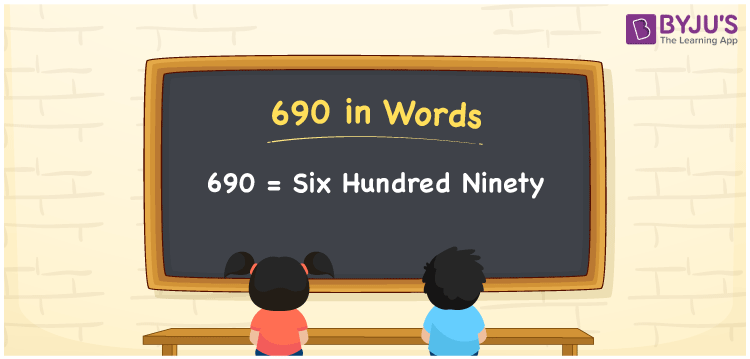# 690 in Words

690 in words is Six hundred ninety. In Mathematics, the number 690 is a natural number and it has three digits. Also, it is a cardinal number, since it defines the specific quantity. For example, the cost of 20 chocolates is Rs. 690. Now, let us discuss how to write the number 690 in words using the place value system in detail.

 690 in Words: Six Hundred Ninety. Six Hundred Ninety in Numerical Form: 690.

## 690 in English Words## How to Write 690 in Words?

The place value table for the number 690 is presented below:

 Hundreds Tens Ones 6 9 0

The expanded form of 690 is as follows:

= 6 × Hundred + 9 × Ten + 0 × One

= 6 × 100 + 9 × 10 + 0 × 1

= 600 + 90

= 690

= Six hundred ninety

Hence, 690 in words is six hundred ninety.

690 in words – Six hundred ninety

Is 690 an odd number? – No

Is 690 an even number? – Yes

Is 690 a perfect square number? – No

Is 690 a perfect cube number? – No

Is 690 a prime number? – No

Is 690 a composite number? – Yes

## Frequently Asked Questions on 690 in Words

Q1

### How to spell 690?

690 in words is six hundred ninety.

Q2

### Simplify 600 + 90, and express it in words.

Simplifying 600 + 90, we get 690. Hence, 690 in words is six hundred ninety.

Q3

### Is 690 an even number?

Yes, 690 is an even number.